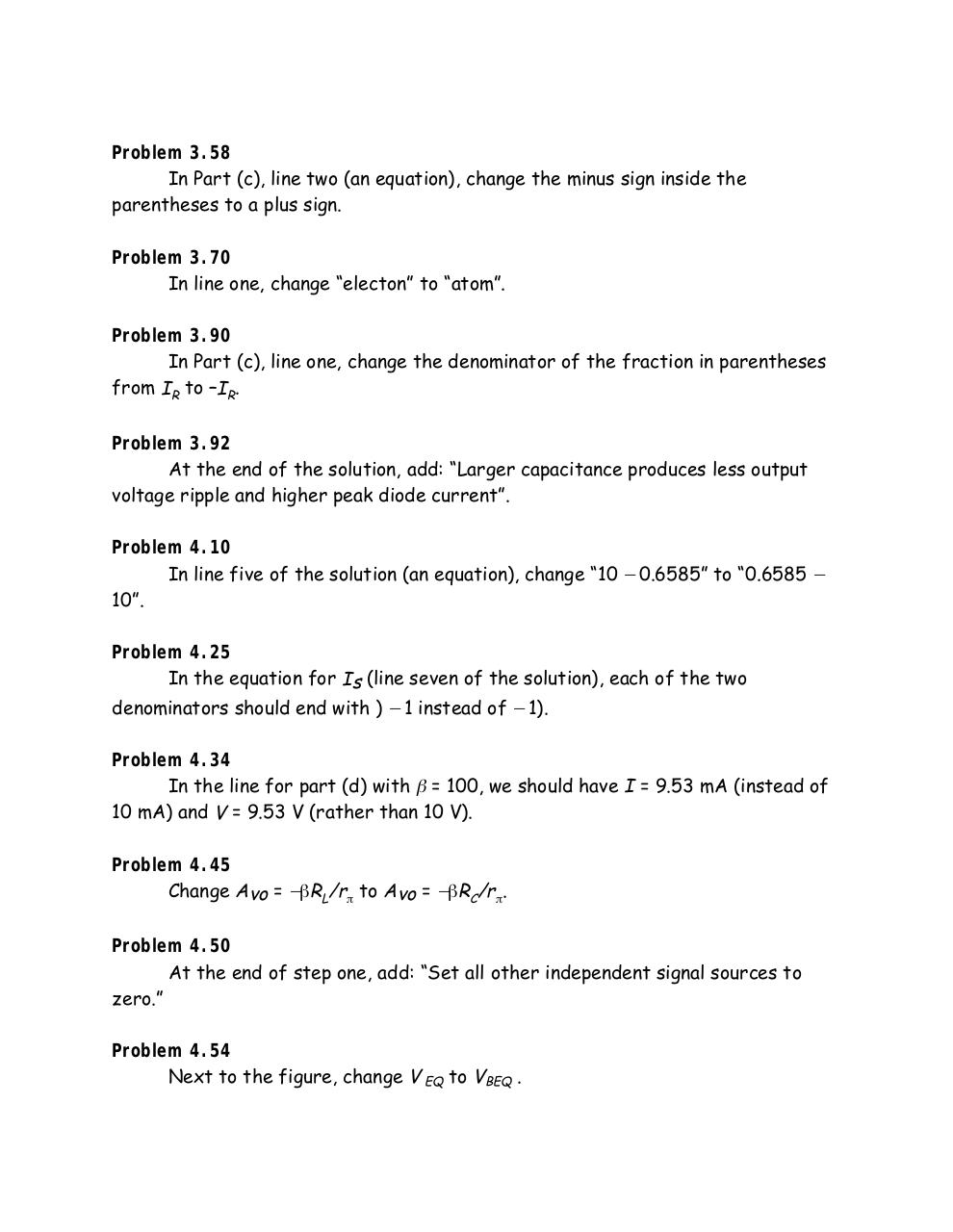# Electronics Hambley 2 edicion.pdfPage 1 2 345561

#### Text preview

Problem 3.58
In Part (c), line two (an equation), change the minus sign inside the
parentheses to a plus sign.
Problem 3.70
In line one, change “electon” to “atom”.
Problem 3.90
In Part (c), line one, change the denominator of the fraction in parentheses
from IR to –IR.
Problem 3.92
At the end of the solution, add: “Larger capacitance produces less output
voltage ripple and higher peak diode current”.
Problem 4.10
In line five of the solution (an equation), change “10 − 0.6585” to “0.6585 −
10”.
Problem 4.25
In the equation for Is (line seven of the solution), each of the two

denominators should end with ) − 1 instead of − 1).

Problem 4.34
In the line for part (d) with β = 100, we should have I = 9.53 mA (instead of
10 mA) and V = 9.53 V (rather than 10 V).
Problem 4.45
Change Avo = −βRL/rπ to Avo = −βRC/rπ.
Problem 4.50
At the end of step one, add: “Set all other independent signal sources to
zero.”
Problem 4.54
Next to the figure, change V EQ to VBEQ .